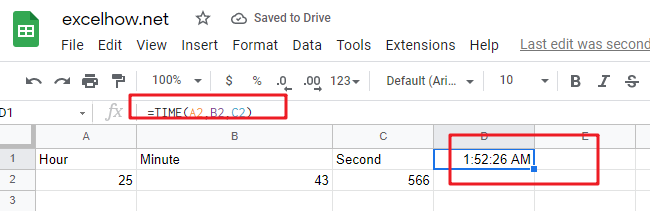This post will guide you how to use Google Sheets TIME function with syntax and examples.

Description

The Google Sheets TIME function converts an hour, minute and second into a time.

The TIME function can be used to create a time with individual hour, minute and second components in google sheets. The purpose of this function is to create a time with the specified hours, minutes and seconds and it also will return a decimal number representing a particular time in google sheets.

The TIME function is a build-in function in Google Sheets and it is categorized as a Date function.

Syntax

The syntax of the TIME function is as below:

= TIME(hour, minute, second)

Where the TIME function arguments are:

• Hour -This is a required argument. A number from 0 (zero) to 32767 representing the hour. If the value is greater than 23 and then it will be divided by 24 and the remainder will be treated as the hour value. For example, the TIME(28,0,0) is equal to TIME(4,0,0).
• Minute – This is a required argument. A number from 0 to 32767 representing the minute. If the minute value is greater than 59 and it will be converted to hours and minutes (it means that every 60 minutes will add one hour to hour value). For example, TIME(1,75,0) = TIME(2,15,0).
• Second – This is a required argument. A number from 0 to 32767 representing the second. If the second value is greater than 59 and it will be converted to hours, minutes, and seconds (it means that every 60 seconds will add 1 minute to minute value). For example, TIME(1,20,75) = TIME(1,21,15) .

Note:

• All of arguments must be numbers and if a string or a cell reference that containing a text string is provided, and TIME function returns #VALUE! Error message.
• TIME function will truncate decimal values of input into the function, for example, an hour component value of 10.58 will be recognized as 10.

The below examples will show you how to use google sheets TIME function to create a decimal number representing a particular time.

#1 =TIME(A2,B2,C2)Related Posts

This post will guide you how to use Google Sheets EXP function with syntax and examples. Description The Google Sheets EXP function returns e raised to the power of a number.  The constant e is equal to 2.71828182845904, and it ...

This post will guide you how to use Google Sheets FACT function with syntax and examples. Description The Google Sheets FACT function returns the factorial of a given number.  The factorial of a given number is equal to 1*2*3…* number. ...

This post will guide you how to use Google Sheets EVEN function with syntax and examples. Description The Google Sheets EVEN function rounds a given number up to the nearest even integer. You can use the EVEN function to return ...

This post will guide you how to use Google Sheets COUNTBLANK function with syntax and examples. Description The Google Sheets COUNTBLANK function use to count the number of empty cells in a range of cells. You can use the COUNTBLANK ...

This post will guide you how to use Google Sheets DEGREES function with syntax and examples. Description The Google Sheets DEGREES function converts radians into degrees. And it will return a numeric value. For examples, if you pass the PI() function into ...

This post will guide you how to use Google Sheets COSH function with syntax and examples. Description The Google Sheets COSH function returns the hyperbolic cosine of a given angle. The purpose of this function is used to get hyperbolic ...

This post will guide you how to use Google Sheets COS function with syntax and examples. Description The Google Sheets COS function returns the cosine of a given angle. If you want to supply an angle to COS function in ...